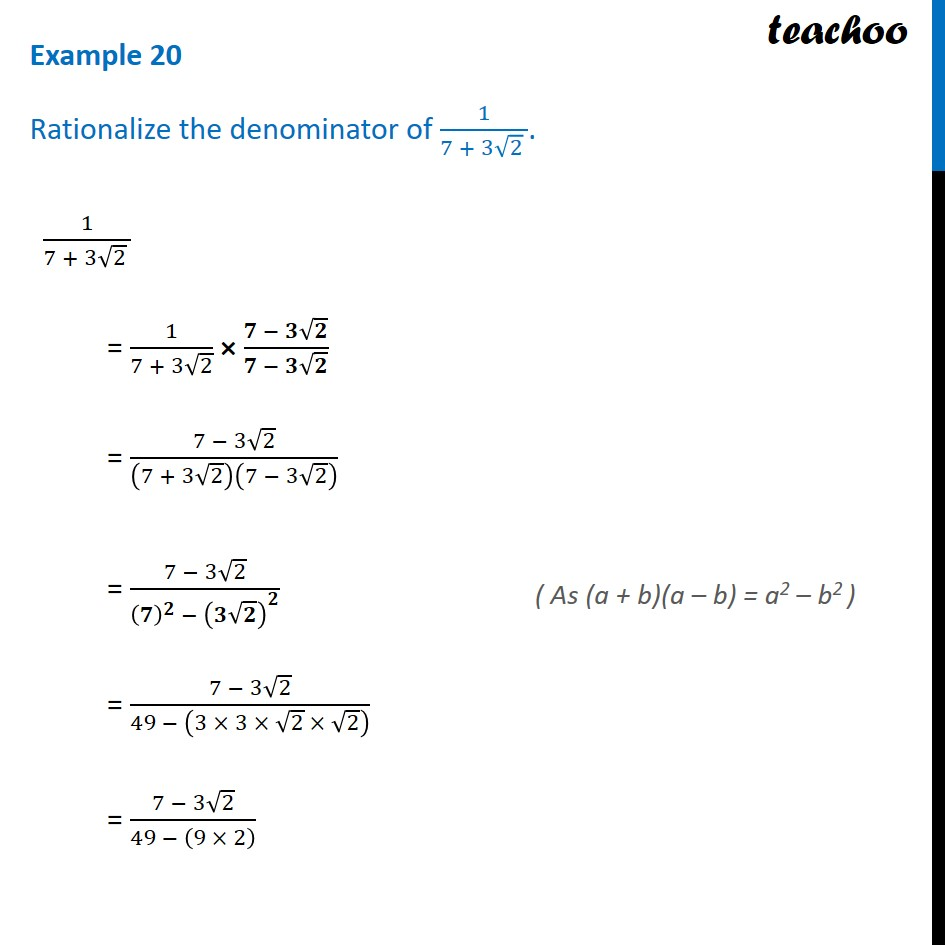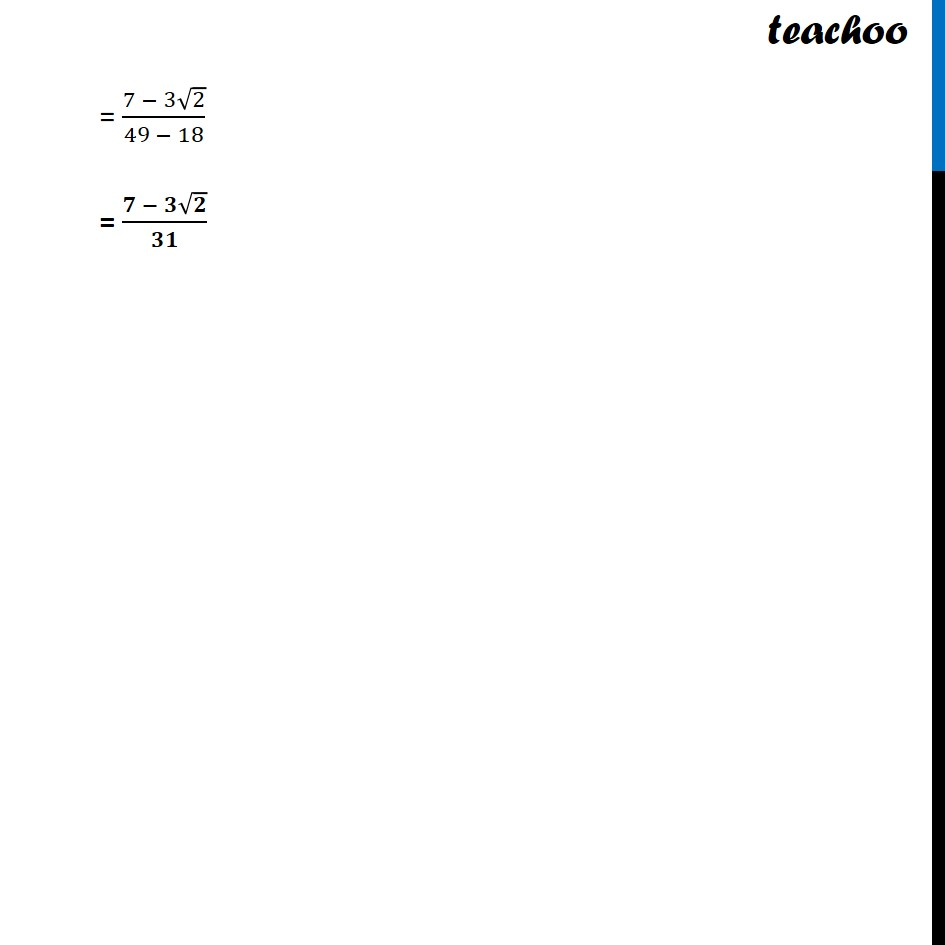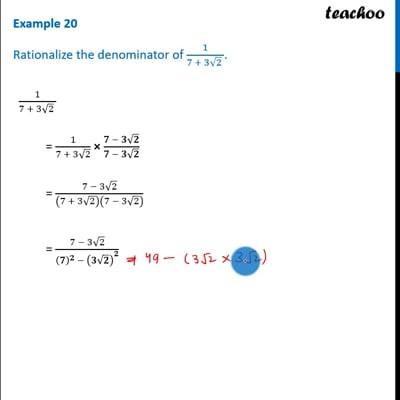Rationalising

Chapter 1 Class 9 Number Systems
Concept wiseThis video is only available for Teachoo black users

Introducing your new favourite teacher - Teachoo Black, at only ₹83 per month

### Transcript

Example 20 Rationalize the denominator of 1/(7 + 3√2 ). 1/(7 + 3√2 ) = 1/(7 + 3√2) × (𝟕 − 𝟑√𝟐)/(𝟕 − 𝟑√𝟐) = (7 − 3√2)/(7 + 3√2)(7 − 3√2) = (7 − 3√2)/((𝟕)^𝟐 − (𝟑√𝟐)^𝟐 ) = (7 − 3√2)/(49 − (3 × 3 × √2 × √2) ) = (7 − 3√2)/(49 − (9 × 2) ) ( As (a + b)(a – b) = a2 – b2 ) = (7 − 3√2)/(49 − 18) = (𝟕 − 𝟑√𝟐)/𝟑𝟏